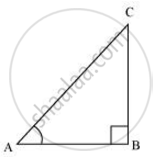Share
Notifications

View all notifications
Books Shortlist
Your shortlist is empty

# State whether the following are true or false. Justify your answer. sec A = 12/5 for some value of angle A. - CBSE Class 10 - Mathematics

Login
Create free account

Forgot password?

#### Question

State whether the following are true or false. Justify your answer.

sec A = 12/5 for some value of angle A.

#### Solution

sec A =  12/5Hypotenuse/Side adjacent to ∠A -  12/5

AC/AB = 12/5

Let AC be 12k, AB will be 5k, where k is a positive integer.

Applying Pythagoras theorem in ΔABC, we obtain

AC2 = AB2 + BC2

(12k)2 = (5k)2 + BC2

144k2 = 25k2 + BC2

BC2 = 119k2

BC = 10.9k

It can be observed that for given two sides AC = 12k and AB = 5k,

BC should be such that,

AC − AB < BC < AC + AB

12k − 5k < BC < 12k + 5k

7< BC < 17 k

However, BC = 10.9k. Clearly, such a triangle is possible and hence, such value of sec A is possible.

Hence, the given statement is true.

Is there an error in this question or solution?

#### APPEARS IN

NCERT Solution for Mathematics Textbook for Class 10 (2019 to Current)
Chapter 8: Introduction to Trigonometry
Ex.8.10 | Q: 11.2 | Page no. 181
Solution State whether the following are true or false. Justify your answer. sec A = 12/5 for some value of angle A. Concept: Trigonometric Ratios.
S